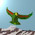## Friday, April 30, 2010

### M Theory Lesson 320

Consider the triple product of three such scaled $R_2$ factors,where $a$, $b$ and $c$ are real. By design, the matrix $M$ is the sum of a real $1$-circulant and an imaginary $2$-circulant. It follows that it is a doubly magic matrix, determined by a $2 \times 2$ block. Consider the block $B$ of the norm squares of $M$ given byAfter renormalising, this is fitted to the canonical $2 \times 2$ block of the CKM matrix (norm squares):The full CKM matrix follows from the magic properties. I used Maxima for this, and a row sum parameter for $|M|^2$ of $192.9986$, which turns out to be close to the natural normalisation of $M$ given by
$(a^2 + 1)(b^2 + 1)(c^2 + 1)$

#### 3 comments:

1.Of course, this does use 4 parameters to fix the small entries of the CKM.

If you look at the complex matrix M more closely, you can see that the scaled values (by roughly sqrt(192)) of a,ab,c are really three real entries from the non squared CKM matrix.

2.That would be the Up row of the CKM matrix. That is, the Up row generates all the other rows.

3.OK, so the best result for the 4 parameter method (ie. with a row sum shift $k$ used to adjust the small values from the 2x2 block) is:

$a = 0.2308985616$
$b = 13.103671706$
$c = -0.065260610$
$k = 181.932270916$

resulting in (all within exp. errors):

$0.974400$, $0.224793$, $0.003584$;
$0.224651$, $0.973556$, $0.041476$;
$0.008737$, $0.040703$, $0.999133$.

Note: Only a member of this blog may post a comment.